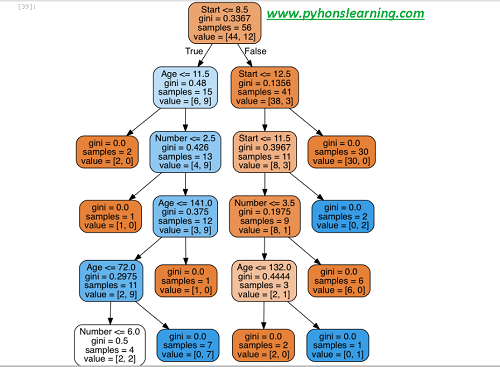# Decision Trees and Random Forests classifier in Python

Welcome everyone, Today we will see Decision Trees and Random Forests classifier-and Types in Python so let's start:
In this project following steps are used to performed operation:
• Import algorithm Decision Trees and Random Forests classifier package.
• Get the data
• Split data into x/y_training and x/y_test data.
• Train or fit the data into the different model methods.
• Prediction and Evaluation the data
• Decision Trees visualization
• Random Forests
• finally generate the Tree (learn different python terminologies)

## Import algorithm Decision Trees and Random Forests classifier package

```

Import Libraries

import pandas as pd

import numpy as np

import matplotlib.pyplot as plt

import seaborn as sns

%matplotlib inline

```

Get the Data
```

```

```

Decision  Age Number Start

0 present 34 3 9

1 absent 58 4 15

2 absent 28 5 8

3 present 72 3 4

4 absent 81 4 15

```

### Split data into x/y_training and x/y_test data.

Let's start to split up the data into a training and test set.
```

from sklearn.model_selection import train_test_split

x = df.drop('Decision Trees',axis=1)

y = df['Decision Trees']

x_train, x_test, y_train, y_test = train_test_split(x, y, test_size=0.20)

```

#### check out Decision Trees

We will start just by training a single decision trees in this section.
```

from sklearn.tree import DecisionTreeClassifier

dtree = DecisionTreeClassifier()

dtree.fit(x_train,y_train)

```
```

DecisionTreeClassifier(class_weight=None, criterion='gini', max_depth=None,

max_features=None, max_leaf_nodes=None, min_samples_leaf=1,

min_samples_split=2, min_weight_fraction_leaf=0.0,

presort=False, random_state=None, splitter='best')

```

How to Prediction and Evaluation the data
Let's start to evaluate our decision tree.
```

predictions = dtree.predict(x_test)

from sklearn.metrics import classification_report,confusion_matrix

print(classification_report(y_test,predictions))

```

```

precisionx    recallx  f1-scorex   supportx

present       0.80      0.80      0.80        15

absent       0.45      0.45      0.45         10

avg / total       0.75      0.75      0.75        25

```

```

print(confusion_matrix(y_test,predictions))

[[18  4]

[ 2  3]

```
Tree Visualization
```

from IPython.display import Image

from sklearn.externals.six import StringIO

from sklearn.tree import export_graphviz

import pydot

```

```

features = list(df.columns[1:])

features

['Ages', 'Numbers', 'Starts']

dot_data = StringIO()

export_graphviz(dtree, out_file=dot_data,feature_names=features,filled=True,rounded=True)

graph = pydot.graph_from_dot_data(dot_data.getvalue())

Image(graph.create_png())

```Decision Trees and Random Forests classifier-Types in Python

Random Forests
Now This time to compare the decision tree model to a random forest.
```

from sklearn.ensemble import RandomForestClassifier

rfc = RandomForestClassifier(n_estimators=100)

rfc.fit(x_train, y_train)

```

```

RandomForestClassifier(bootstrap=True, class_weight=None, criterion='gini',

max_depth=None, max_features='auto', max_leaf_nodes=None,

min_samples_leaf=1, min_samples_split=2,

min_weight_fraction_leaf=0.0, n_estimators=100, n_jobs=1,

oob_score=False, random_state=None, verbose=0,

warm_start=False)

rfc_pred = rfc.predict(X_test)

```

```

print(confusion_matrix(y_test,rfc_pred))

[[14  3]

[ 2  4]]

print(classification_report(y_test,rfc_pred))

```

```

precisionx    recallx  f1-scorex   supportx

present     0.83      0.80      0.78        10

absent      0.55      0.50      0.54         15

avg / total       0.75      0.70      0.77        25

```
How many types of decision trees in machine learning?
It's based on the type of target variable in python.
1. Categorical variable Decision Tree: If in Decision Tree which has a available categorical target variable then it called a Categorical variable decision tree in python.
Summary:
In this section we saw the Decision Trees and Random Forests classifier-Types in Python. About this section you have any query then please comment
Tags:
Decision Trees and Random Forests classifier-Types in Python, python, machine learning
If you want to machine learning interview question then click on it.
BEST OF LUCK!!!

Previous
Next Post »

## Featured Snippet

### 10 ways to iterate through a list in python

10 ways to iterate through a list in python The list is similar to array in other languages except for python, which provides the extra b...